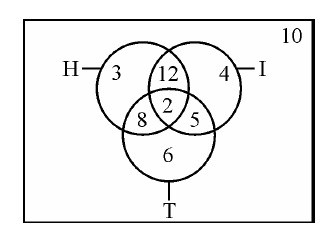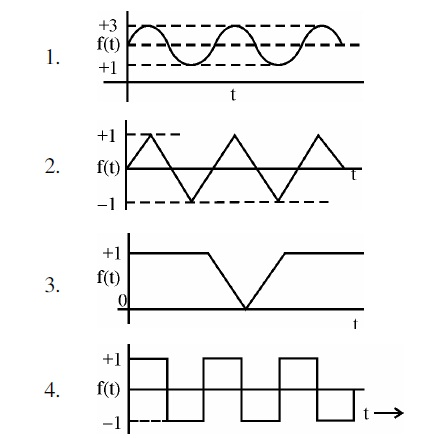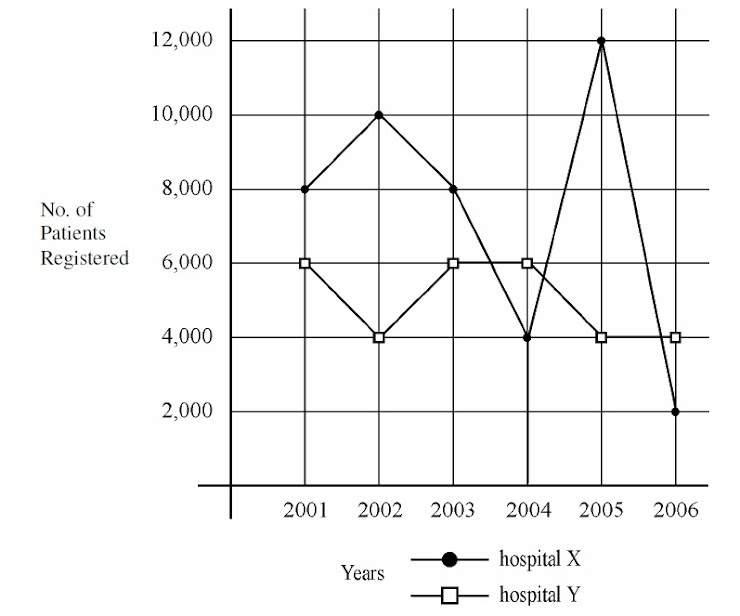UGC-NET/SET-PAPER-I(2009-DEC) - MODEL PAPER-93
ONLINE TEST NET/SET PAPER-1
Email address *
31. Deductive argument involves
2 points
32. Inductive reasoning is based on or presupposes
2 points
33. To be critical, thinking must be
2 points
34. Which of the following is an analogous statement?
2 points
Questions from 35 - 36 are based on the following diagram in which there are three intersecting circles. H representing The Hindu, I representing Indian Express and T representing The Times of India. A total of 50 persons were surveyed and the number in the Venn diagram indicates the number of persons reading the newspapers.
2 points36. How many persons would be reading almost two newspapers?
2 points
37. Which of the following graphs does not represent regular (periodic) behavior of the variable f(t)?
2 pointsStudy the following graph and answer the questions 38 to 40:
2 points39. What is the maximum dispersion in the registration of patients in the two hospitals in a year?
2 points
40. In which year there was maximum decrease in registration of patients in hospital X?
2 points